Study Materials: CBSE Notes

Our CBSE Notes for Assignments Class 9 maths 10. Circles - CBSE Notes - Toppers Study is the best material for English Medium students cbse board and other state boards students.

Notes ⇒ Class 9th ⇒ Mathematics ⇒ 10. Circles

# Assignments Class 9 maths 10. Circles - CBSE Notes - Toppers Study

Topper Study classes prepares CBSE Notes on practical base problems and comes out with the best result that helps the students and teachers as well as tutors and so many ecademic coaching classes that they need in practical life. Our CBSE Notes for Assignments Class 9 maths 10. Circles - CBSE Notes - Toppers Study is the best material for English Medium students cbse board and other state boards students.

## Assignments Class 9 maths 10. Circles - CBSE Notes - Toppers Study

CBSE board students who preparing for class 9 ncert solutions maths and Mathematics solved exercise chapter 10. Circles available and this helps in upcoming exams 2023-2024.

### You can Find Mathematics solution Class 9 Chapter 10. Circles

• All Chapter review quick revision notes for chapter 10. Circles Class 9
• NCERT Solutions And Textual questions Answers Class 9 Mathematics
• Extra NCERT Book questions Answers Class 9 Mathematics
• Importatnt key points with additional Assignment and questions bank solved.

NCERT Solutions do not only help you to cover your syllabus but also will give to textual support in exams 2023-2024 to complete exercise maths class 9 chapter 10 in english medium. So revise and practice these all cbse study materials like class 9 maths chapter in english ncert book. Also ensure to repractice all syllabus within time or before board exams for ncert class 9 maths ex in english.

See all solutions for class 9 maths chapter 10 exercise 10 in english medium solved questions with answers.

### Assignments class 9 Mathematics Chapter 10. Circles

• Assignments Class 9 Maths 10. Circles - CBSE Notes - Toppers Study
• Class 9 Ncert Solutions
• Solution Chapter 10. Circles Class 9
• Solutions Class 9
• Chapter 10. Circles Assignments Class 9

## Assignments Class 9 maths 10. Circles - CBSE Notes - Toppers Study

### Important-Question for Assignment:

Q1. The bisector of ∠B of an isosceles triangle ABC with AB = Ac meets the circumcircle of  ABC at P as shown in figure. If AP abd BC produced meet at Q, prove that CQ = CA.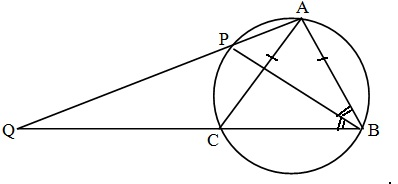Q2. In the given figure, O is the centre of the circle. The angle substended by arc ABC at the centre is 140 , AB is produced to P. Determine ∠ADC and ∠CBP.Q3. In the given figure, O is the centreand Ae is the semi-circle ABCDE. If AB = BC and ∠AEC = 50o, then find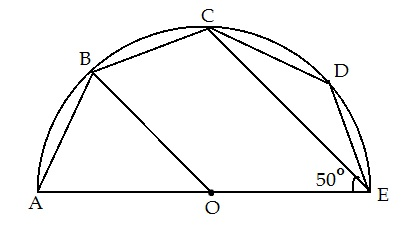(i) ∠CBE

(ii) ∠CDE

(iii) ∠AOB

​(iv) Prove that BO|| CE

Q4.  In figure, P is the centre of  the circle. Prove that

∠XPZ = 2 (∠XZY + ∠YXZ)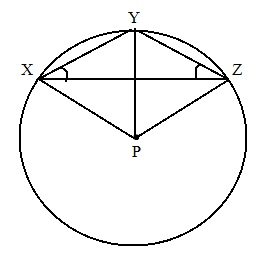Q5. In given fig. B and E are the midpoint on respectively on line segment AC and DF. Show that AD||CF.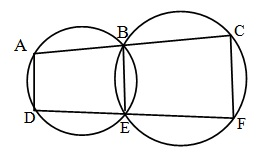Q6. Find the value of x see given figure.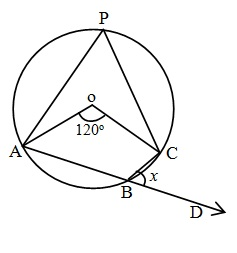Q7.  ABCD is a parallelogram. The circle through A, B and C intersects CD produced at E, prove that AE = AD.Q8. In the given Figure, find the value of x.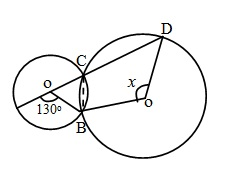Q9. L and M are the mid-point of two equal chords AB and CD and O is the centre of the circle. Prove that

(i) OLM = OML

(ii) ALM = CML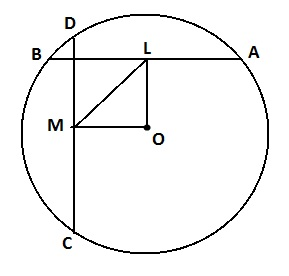##### Other Pages of this Chapter: 10. Circles

Important Study materials for classes 06, 07, 08,09,10, 11 and 12. Like CBSE Notes, Notes for Science, Notes for maths, Notes for Social Science, Notes for Accountancy, Notes for Economics, Notes for political Science, Noes for History, Notes For Bussiness Study, Physical Educations, Sample Papers, Test Papers, Mock Test Papers, Support Materials and Books.Mathematics Class - 11th

NCERT Maths book for CBSE Students.

books

## Study Materials List:

##### Notes ⇒ Class 9th ⇒ Mathematics
1. Number Systems
2. Polynomials
3. Coordinate Geometry
4. Linear Equation In Two Variables
5. Introduction To Euclid’s Geometry
6. Lines and Angles
7. Triangles